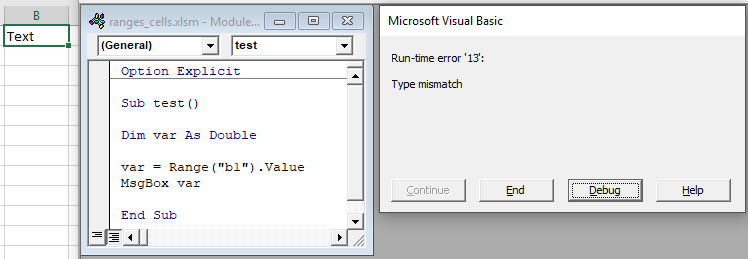### VBA Cell Value – Get, Set, or Change

This tutorial will teach you how to interact with Cell Values using VBA.

## Set Cell Value

To set a Cell Value, use the Value property of the Range or Cells object.

### Range.Value & Cells.Value

There are two ways to reference cell(s) in VBA:

• Range Object – Range(“A2”).Value
• Cells Object – Cells(2,1).Value

The Range object allows you to reference a cell using the standard “A1” notation.

This will set the range A2’s value = 1:

The Cells object allows you to reference a cell by it’s row number and column number.

This will set range A2’s value = 1:

Notice that you enter the row number first:

### Set Multiple Cells’ Values at Once

Instead of referencing a single cell, you can reference a range of cells and change all of the cell values at once:

### Set Cell Value – Text

In the above examples, we set the cell value equal to a number (1).  Instead, you can set the cell value equal to a string of text.  In VBA, all text must be surrounded by quotations:

If you don’t surround the text with quotations, VBA will think you referencing a variable…

### Set Cell Value – Variable

You can also set a cell value equal to a variable

## Get Cell Value

You can get cell values using the same Value property that we used above.

### Get ActiveCell Value

To get the ActiveCell value and display it in a message box:

### Assign Cell Value to Variable

To get a cell value and assign it to a variable:

Here we used a variable of type Variant. Variant variables can accept any type of values.  Instead, you could use a String variable type:

A String variable type will accept numerical values, but it will store the numbers as text.

If you know your cell value will be numerical, you could use a Double variable type (Double variables can store decimal values):

However, if you attempt to store a cell value containing text in a double variable, you will receive an error:## Other Cell Value Examples

### Copy Cell Value

It’s easy to set a cell value equal to another cell value (or “Copy” a cell value):

You can even do this with ranges of cells (the ranges must be the same size):

### Compare Cell Values

You can compare cell values using the standard comparison operators.

Test if cell values are equal:

Will return TRUE if cell values are equal. Otherwise FALSE.

You can also create an If Statement to compare cell values:

You can compare text in the same way (Remember that VBA is Case Sensitive)